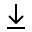MathBin Percent Member

Percent or percentage is a number or ratio expressed as a fraction of 100. It is often denoted using the percent sign, "%". A percentage is a dimensionless number (pure number). For example, 45% (read as "forty-five percent") is equal to 45/100, or 0.45.

Bins